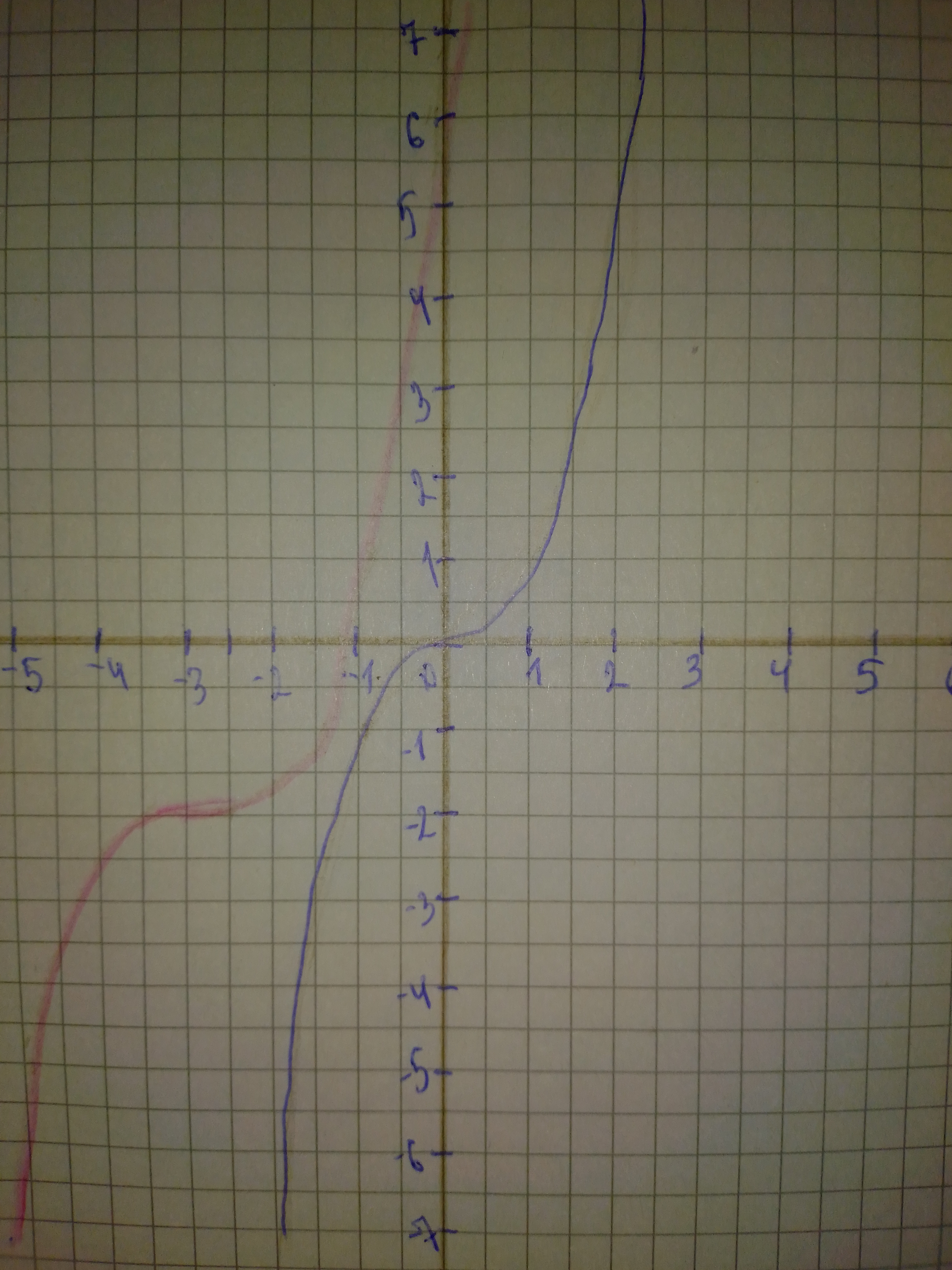# In calculus, it can be shown that the arctangent function can be approximated by the polynomial arctan x approx x-x^{3}/3+x^{5}/5-x^{7}/7 where x is icoexpennan 2021-02-25 Answered
In calculus, it can be shown that the arctangent function can be approximated by the polynomial
$\mathrm{arctan}x\approx x-\frac{{x}^{3}}{3}+\frac{{x}^{5}}{5}-\frac{{x}^{7}}{7}$
where x is in radians. Use a graphing utility to graph the arctangent function and its polynomial approximation in the same viewing window. How do the graphs compare?
You can still ask an expert for help

• Questions are typically answered in as fast as 30 minutes

Solve your problem for the price of one coffee

• Math expert for every subject
• Pay only if we can solve itsaiyansruleA

Step 1
The red graph is arctanx and the blue graph is the approximation. They are similar close to the origin.